Author: Oscar Cronquist Article last updated on February 02, 2018

This calendar lets you schedule events on the data sheet. Select a cell (calendar date) and events on that day are shown below the calendar.

Today´s date is highlighted yellow. Days with one or more events are also highighted.

### Drop down lists

Select year

1. Select cell B2
2. Go to tab "Data"
3. Click "Data Validation" button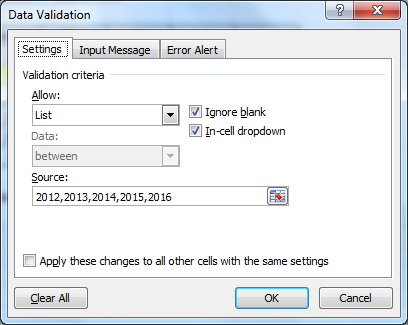4. Allow: List
5. Source:2012,2013,2014,2015,2016
6. Click OK

Select month

1. Select cell E4
2. Go to tab "Data"
3. Click "Data Validation" button
4. Allow: List
5. Source:January, February, March, April, May, June, July, August, September, October, November, December
6. Click OK

Type Year: in cell B2, Month: in cell E2 and so on...

### Calculating dates (formula)

1. Select cell B5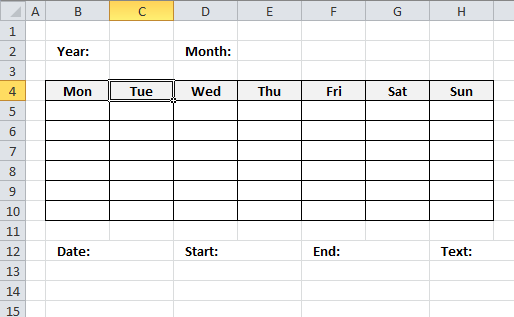2. Formula:
=DATE(\$C\$2,MATCH(\$E\$2,{"January"; "February"; "March"; "April"; "May"; "June"; "July"; "August"; "September"; "October"; "November"; "December"},0),1)-WEEKDAY(DATE(\$C\$2,MATCH(\$E\$2,{"January"; "February"; "March"; "April"; "May"; "June"; "July"; "August"; "September"; "October"; "November"; "December"},0),1),2)+1
3. Select cell B6
4. Formula:
=B5+1
5. Copy cell B6 (Ctrl + c)
6. Select cell range D5:H5
7. Paste (Ctrl + v)
8. Select cell B6
9. Formula:
=B5+7
10. Copy cell range C5:H5
11. Select cell range C6:H6
12. Paste (Ctrl + v)
13. Copy cell range B6:H6 (Ctrl + c)
14. Select cell range B7:H10
15. Paste (Ctrl + v)

### Conditional formatting

Highlight Today

1. Select cell range B5:H10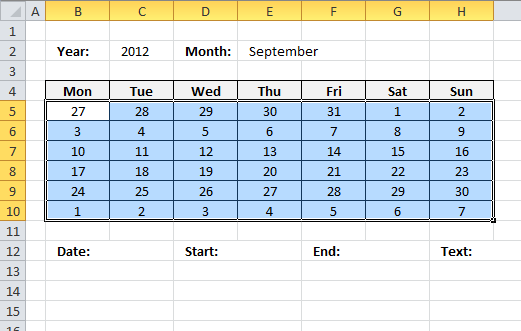2. Go to tab "Home"
3. Click "Conditional formatting" button
4. Click "New Rule..."
5. Click "Use a formula to determine which cells to format"
6. Format values where this formula is true:
=B5=TODAY()
7. Click "Format..." button
8. Go to "Fill" tab
9. Pick a color
10. Click OK
11. Click OK

Change font color for dates not in selected month

1. Repeat 1-5 steps above
2. =MONTH(B5)<>MONTH(\$B\$6)
3. Click "Format..." button
4. Go to "Font" tab
5. Pick a color
6. Click OK
7. Click OK

Highlight days with events

1. Repeat 1-5 steps above
2. =OR(B5=INT(INDEX(Data,0,1)))
3. Click "Format..." button
4. Go to "Fill" tab
5. Pick a color
6. Click OK
7. Click OK

### Formulas

Dynamic named range

1. Select sheet "Data"
2. Go to tab "Formulas"
3. Click "Name Manager" button
4. Click "New..."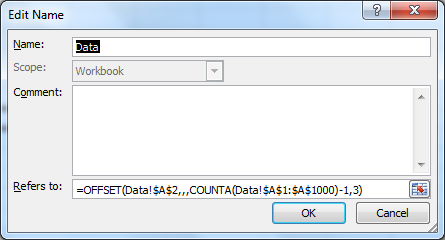5. Name: Data
6. Refers to:
=OFFSET(Data!\$A\$2,,,COUNTA(Data!\$A\$1:\$A\$1000)-1,3)
7. Click OK!
8. Click Close

Event formulas

1. Select sheet "Calendar"
2. Select cell B13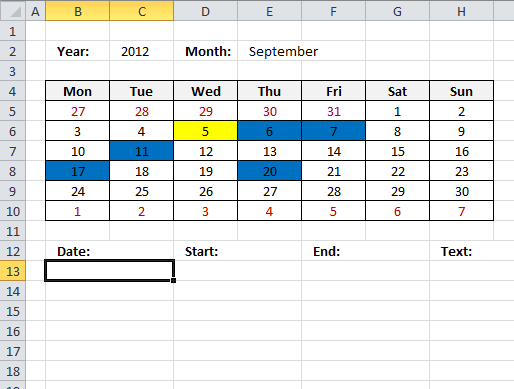3. Array formula:
=IFERROR(INT(INDEX(Data, SMALL(IF(INT(INDEX(Data, 0, 1))=\$G\$2, MATCH(ROW(Data), ROW(Data)), ""), ROW(A1)), COLUMN(A1))), "")
4. How to create an array formula
5. Select cell D13
6. Array formula:
=IFERROR(INDEX(Data, SMALL(IF(INT(INDEX(Data, 0, 1))=\$G\$2, MATCH(ROW(Data), ROW(Data)), ""), ROW(B1)), COLUMN(A1)), "")
7. Select cell F13
8. Array formula:
=IFERROR(INDEX(Data, SMALL(IF(INT(INDEX(Data, 0, 1))=\$G\$2, MATCH(ROW(Data), ROW(Data)), ""), ROW(C1)), COLUMN(B1)), "")
9. Select cell H13
10. Array formula:
=IFERROR(INDEX(Data, SMALL(IF(INT(INDEX(Data, 0, 1))=\$G\$2, MATCH(ROW(Data), ROW(Data)), ""), ROW(D1)), COLUMN(C1)), "")

How to create an array formula

1. Select a cell
2. Click in formula bar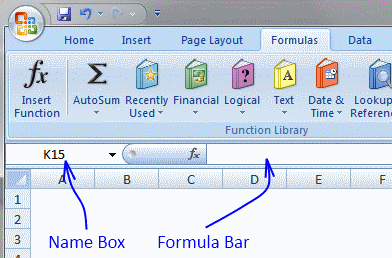3. Paste array formula
4. Press and hold Ctrl + Shift
5. Press Enter

### Visual basic for applications

1. Right click on Calendar sheet
2. Click "View code"
3. Copy vba code
```Private Sub Worksheet_SelectionChange(ByVal Target As Range)
If Not Intersect(Target, Range("B5:H10")) Is Nothing Then
Range("G2") = Target.Value
End If
End Sub
```
4. Paste to code module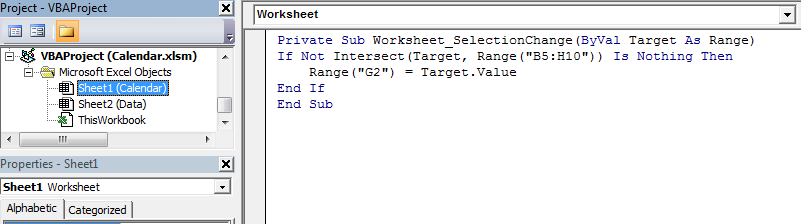Hide value in cell G2

1. Select cell G2
2. Press Ctrl + 1
3. Go to tab "Number"
4. Select category: Custom
5. Type ;;;
6. Click OK!

Calendar.xlsm

### Functions in this post:

INDEX(array, row_num, [column_num])
Returns a value or reference of the cell at the intersection of a particular row and column, in a given range.

MATCH(lookup_value, lookup_array, [match_type])
Returns the relative position of an item in an array that matches a specified value in a specific order

SMALL(array, k)
Returns the k-th smallest value in a data set.

ROW(reference)
Returns the row number of a reference.

IFERROR(value, value_if_error)
Returns value_if_error if expression is an error and the value of expression itself otherwise.